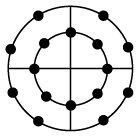# ISRO Scientist or Engineer Electronics 2012

Instructions

For the following questions answer them individually

Question 21

# For identical total transmitted power, the ratio of $$\frac{S}{N}$$ for wide band FM to $$\frac{S}{N}$$ of DSB-AM (with $$m_a = 1$$)is :( Given fm = 15 KHz & frequency.deviation =$$\pm$$ 75 KHz)Question 22

# A signal $$m(t) = 3 \cos(6000 \pi t) + 4 \cos(7000 \pi t) + 5 \cos(10000 \pi t)$$ to be sampled. What is the minimum sampling rate for band pass sampling for truth full representation :Question 23

# Minimum number of bits required to represent maximum value of given analoguesignal with 0.1% accuracyis:Question 24

# An Ideal powerlimited communication channel with additive white Gaussian noise is having 4 KHz band width and Signal to Noise ratio of 255. The channel capacityis:Question 25

# An Ideal power limited communication channel with additive white Gaussian noise with 1 MHz band width and Signal to Noise ratio of 15 is transmitting the | information at theoretical maximum rate. If the Signal to Noise ratio is reduced to 7, how much bandwidthis required to maintain samerate:Question 26

# Following constellation diagram represents:Question 27

# The information rate to be transmitted is 900 bits /sec, FEC is $$\frac{3}{4}$$ , the symbol rate for QPSK modulation scheme is:Question 28

# Electric displacement density D at any point on spherical surface of radius R with a charge Q at the centre ina medium with dielectric constant $$\epsilon$$ is:Question 29

# Which of the following does not represent Maxwell’s Equation:Question 30

# For a homogeneous and isotropic medium with a charge density $$\rho$$ & dielectric constant $$\epsilon$$, the Poisson’sequation for electrical potential V is :OR